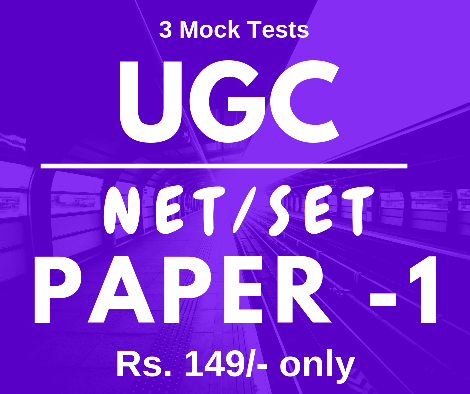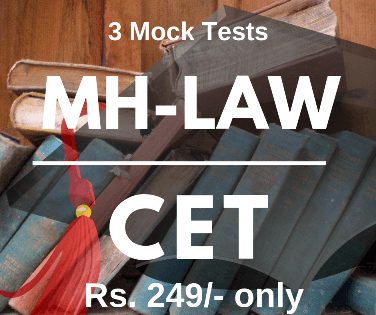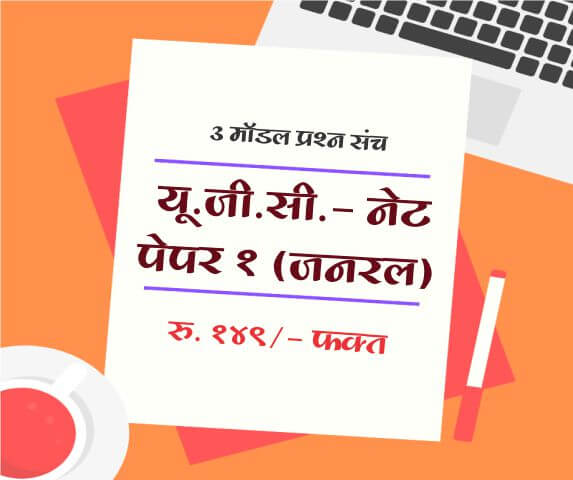# Understanding Profit & Loss problems with Examples

1 Basic Definitions

Profit and Loss is important topic in Quantative Aptitude test, lets see basic definition of Profit and Loss.

The price at which articles/ item purchased is called as Cost Price (C.P.).

The price at which articles/ item sold is called as Selling Price (S.P.).

2 Profit or Gain

If Selling Price (S.P.) is more than the Cost Price (C.P.) of article/ item then the difference between selling price and cost price is known as Profit.

Formula for % Gain is as follows

Formula can be also represented as follows

Formula for calculating Selling Price (S.P.) when % Gain/Profit and Cost Price (C.P.) is given for article.

Formula for calculating Cost Price (C.P.) when % Gain/Profit and Selling Price (S.P.) is given for article.

Profit Calculator
3 Loss

If Selling Price (S.P.) is less than the Cost Price (C.P.) of article/ item then the difference between selling price and cost price is known as Loss.

Formula for % Gain is as follows

Formula can be also represented as follows

Formula for calculating Selling Price (S.P.) when % Loss and Cost Price (C.P.) is given for article.

Formula for calculating Cost Price (C.P.) when % Loss and Selling Price (S.P.) is given for article.

Loss Calculator
4 Profit and Loss: Solved Examples

Question 1 Lentil is purchased for Rs. 115 and sold for Rs. 135. Find the profit percent.

Question 2 A shop sold a washing machine for Rs. 14999. Find the cost price if he incurred a loss of 5%.

Question 3 In a transaction, the profit percentage is 60% of the cost. If the cost further increases by 20% but the selling price remain the same, how much is the decrease in profit percentage?

 Name : Comment :

Subscribe to get latest updates about Exam Schedules, Government Jobs, General Knowledge & Current Affairs and Many more on Whatsapp!###### Aptitude

Full Length Mock Tests
Timer Based Exams
Instant Result and assesment
Detailed analasys of Result##### UGC NET SET Paper 1 Mock Tests

UGC-NET / SET Paper 1 mock test

3 Mock Tests, 150 Questions with Explanations

Rs: 149 Only/-##### LAW MH-CET 2019 (3 YEAR COURSE)

Mock test for MH CET Law 2019 for three year law programme

3 Mock Tests, 450 Questions with Explanations

Rs: 249 Only/-##### यू. जी. सी. - नेट पेपर १ (जनरल)

यू. जी. सी. - नेट पेपर १ (अनिवार्य) सामान्य प्रश्न हिंदीमें

3 Mock Tests, 150 Questions with Explanations

Rs: 149 Only/-